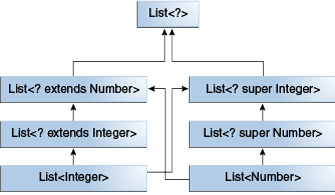## 为什么需要泛型

Generics 给类，接口和方法提供了一个参数化的实现方式，使得同一个类定义，方法定义可以处理不同的类型。Oracle 官方的文档有一句话说得特别好：

Much like the more familiar formal parameters used in method declarations, type parameters provide a way for you to re-use the same code with different inputs.

Java 编译期会在编译阶段检查类型。

• 在编译时进行类型检查，减少错误
• 消除了强制类型装换
• 可以让编程人员更加容易实现通用算法

## 定义

``````class name<T1, T2, ..., Tn> { /* ... */ }
``````

### Type Parameter 命名约定

• `E` for `Element` 被 Java Collections 框架广泛使用
• `K` for `Key` ， K V 经常被 Java Collections 框架中的 Map 使用
• `N` for `Number`
• `T` for `Type`
• `V` for `Value`
• `S,U,V etc` - 2nd, 3rd, 4th types

### 调用和实例化泛型类型

``````List<String> list;
``````

Type Parameter 和 Type Argument 术语：大部分情况下这两个术语是可以互换的，但他们的使用场景是不一样的。因此 `Foo<T>` 中的 T 是 type Parameter，而 `Foo<String>` 中的 String 是 type argument.

## 泛型使用

### 泛型方法

``````public static <K, V> boolean compare(Pair<K, V> p1, Pair<K, V> p2) {...}
``````

``````boolean same = Util.<Integer, String>compare(p1, p2);
// or
boolean same = Util.compare(p1, p2);
``````

### 泛型类

``````class GenericClass<ID, T> {}

class SubGenericClass<T> extends GenericClass<Integer, T> {}
``````

### 泛型接口

``````interface GenericInterface<T> {
T append(T seg);
}
``````

## 有界类型参数

``````<T extends B1 & B2 & B3>
``````

## 通配符

### Upper Bounded Wildcards

``````public static void process(List<? extends Foo> list) { /* ... */ }
``````

upper bounded wildcard，`List<? extends Foo>` 其中 Foo 是类型，表示 Foo 和任何子类。

### Unbounded Wildcards

• 当写方法只需要 `Object` 类中的方法时
• 在泛型类中定义的方法不依赖于 type parameter 时，比如 List.size() 或者 List.clear ，并不依赖于定义的泛型类型

``````public static void printList(List<Object> list) {
for (Object elem : list)
System.out.println(elem + " ");
System.out.println();
}
``````

``````public static void printList(List<?> list) {
for (Object elem: list)
System.out.print(elem + " ");
System.out.println();
}
``````

### Lower Bounded Wildcards

``````public static void addNumbers(List<? super Integer> list) {
for (int i = 1; i <= 10; i++) {
}
}
``````

``````class A { /* ... */ }
class B extends A { /* ... */ }
``````

``````B b = new B();
A a = b;
``````

``````List<B> listB = new ArrayList<>();
List<A> listA = listB;        // 编译错误
``````

`List<Number>``List<Integer>` 都是 `List<?>` 的子类型，而 `List<Number>``List<Integer>` 这两者并没有任何关系。

``````List<? extends Integer> intList = new ArrayList<>();
List<? extends Number>  numList = intList;  // OK. List<? extends Integer> is a subtype of List<? extends Number>
``````## 类型擦除

• 如果类型参数是无界的，则将泛型类型中的所有类型参数替换为其边界或对象。因此，生成的字节码仅包含普通的类，接口和方法。
• 在合适的位置插入类型转换，以保证类型安全
• 生成桥接方法以保留扩展泛型类型中的多态性

Java 编译器还会擦除泛型方法参数中的类型参数。比如静态方法：

``````// Counts the number of occurrences of elem in anArray.
//
public static <T> int count(T[] anArray, T elem) {
int cnt = 0;
for (T e : anArray)
if (e.equals(elem))
++cnt;
return cnt;
}
``````

``````class User implements Comparable<User> {
String name;

public int compareTo(User other){
return this.name.compareTo(other.name);
}
}
``````

JDK 中接口定义：

``````package java.lang;
public interface Comparable<T>{
int compareTo(T o);
}
``````

``````public interface Comparable
{

public abstract int compareTo(Object obj);
}
``````

``````javac User.java
``````

``````jad User.class
``````

``````class User
implements Comparable
{

User()
{
}

public int compareTo(User user)
{
return name.compareTo(user.name);
}

// 桥接方法
public volatile int compareTo(Object obj)
{
return compareTo((User)obj);
}

String name;
}
``````

## 泛型的限制

### 不能使用原始类型来实例化泛型

``````Pair<int, char> p = new Pair<>(8, 'a');  // compile-time error
``````

### 无法创建类型参数的实例

``````public static <E> void append(List<E> list) {
E elem = new E();  // compile-time error
}
``````

``````public static <E> void append(List<E> list, Class<E> cls) throws Exception {
E elem = cls.newInstance();   // OK
}
``````

``````List<String> ls = new ArrayList<>();
append(ls, String.class);
``````

### 无法申明类型参数为静态

``````public class MobileDevice<T> {
private static T os;

// ...
}
``````

### 不能对 parameterized types 进行类型装换和使用 instanceof

``````public static <E> void rtti(List<E> list) {
if (list instanceof ArrayList<Integer>) {  // compile-time error
// ...
}
}
``````

``````public static void rtti(List<?> list) {
if (list instanceof ArrayList<?>) {  // OK; instanceof requires a reifiable type
// ...
}
}
``````

### 无法创建参数化类型的数组

``````List<Integer>[] arrayOfLists = new List<Integer>;  // compile-time error
``````

### 无法创建，捕获或抛出参数化类型的对象

``````// Extends Throwable indirectly
class MathException<T> extends Exception { /* ... */ }    // compile-time error

// Extends Throwable directly
class QueueFullException<T> extends Throwable { /* ... */ // compile-time error
``````

``````class Parser<T extends Exception> {
public void parse(File file) throws T {     // OK
// ...
}
}
``````

### 不能有重载的方法使用相同的泛型

``````public class Example {
public void print(Set<String> strSet) { }
public void print(Set<Integer> intSet) { }
}
``````